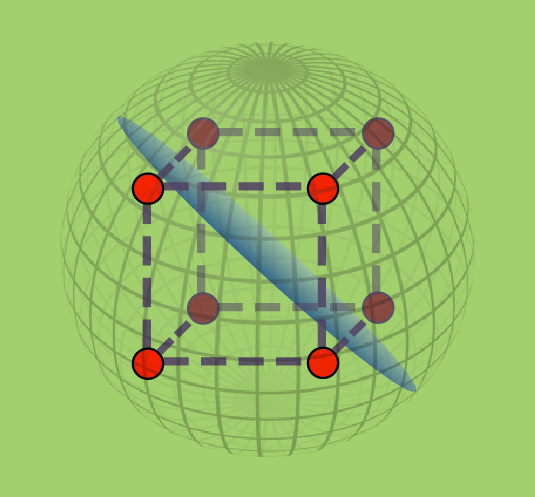# Inapproximability of Constraint Satisfaction Problems#### This series of talks was part of the Real Analysis Boot Camp. Videos for each talk are available through the links above.

Speaker: Johan Håstad, KTH Royal Institute of Technology
Starting from the PCP-theorem (which we will take as given) we show how to design and analyze efficient PCPs for NP-problems. We describe how an efficient PCP using small amounts of randomness can be turned into an inapproximability result for a maximum constraint satisfaction problem where each constraint corresponds to the acceptance criterion of the PCP. We then discuss how to design efficient PCPs with perfect completeness in some interesting cases like proving the hardness of approximating satisfiable instances of 3-Sat.

We go on to discuss gadget construction and how to obtain optimal reductions between approximation problems. We present Chan's result on how to take products of PCPs to get hardness for very sparse CSPs and give some preliminary new results using these predicates as a basis for a gadget reduction.

Finally we discuss approximation in a different measure, and in particular the following problem. Given a (2k+1)-CNF formula which admits an assignment that satisfies k literal in each clause, is it possible to efficiently find a standard satisfying assignment?